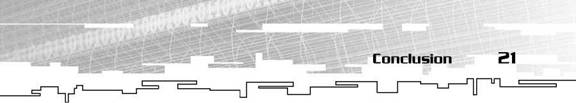# 3Д БУМ

## 3Д принтеры и всё что с ними связано

ЗД принтеры АБС пластик

# The CD’s Code

Take a look at the following directory on the CD: /Code/Chapterl/ or /Code/Math. Here, you will find C++ classes to store and manipulate matrices and vectors. There are separate classes for 3×3 and 4×4 matrices, both defined and implemented in the files matrix. cpp and matrix. inl. The same goes for vectors. There are classes for both 2D and 3D vectors, defined and implemented in vector. h and vector. inl. All of the classes contain a variety of functions to perform the opera­tions covered here, as well as overloaded operators that can be used to do the basic mathematical operations such as addition and subtrac­tion. There are even operators to compare two matrices or vectors and tell you if they are equal. All these operators allow you to use matrices and vectors as if you were just using real numbers. Syntax such as vectorl = vector2 + vector3 and if(matrix1 == matrix2) are both valid thanks to the wonderful features of C++ that allow programmers to override the default definition of the mathematical operators.

In both directories you will notice a few extra files, as follows:

■ math. h is the main header file that basically includes all of the other files, ensuring that you get all of the functionality avail­able, without needing to remember to include a bunch of files.■ The other two files, quaternion. h and quaternion. inl, are the definitions and implementation of a class that is used to store and manipulate quaternions, which you will be learning about in the next chapter. There are a few functions in the matrix and vector classes that use quaternions, generally signified by taking either a reference to a CQuaternion class as a parameter, or return­ing a CQuaternion class. Don’t panic if you don’t know what these functions are for; you will learn about them in the next chapter.

Conclusion

That concludes the brief review of matrix and vector math. If you need or want to learn more about either, I suggest that you visit a local college library or bookstore and pick yourself up a book on linear algebra. The books I used as a reference for most of this were Matrices, written by Frank Ayres Jr. and Linear Algebra, by the same author. Both are part of a series of math references known as Schaum’s Outlines Series of Mathematics. If you cannot afford a book, or simply want a different solution, I once again suggest Eric Weisstein’s World of Math­ematics, available at http://mathworld. wolfram. com. Eric Weisstein’s World of Mathematics has examples and explanations for many mathematical problems, including matrices and vectors.

The next chapter introduces you to quaternions. Quaternions are used by graphics programmers to represent rotations, much like a 3×3 rotation matrix. However, quaternions hold several advantages over traditional matrices, such as the capability to avoid gimbal lock, and the capability to create easy, smooth interpolation between orientations.

To top it all off, they take up less space than matrices. Check it out!